## At a certain time a particle had a speed of 46 m/s in the positive x direction, and 9.1 s later its speed was 67 m/s in the opposite directi

Question

At a certain time a particle had a speed of 46 m/s in the positive x direction, and 9.1 s later its speed was 67 m/s in the opposite direction. What was the average acceleration of the particle during this 9.1 s interval

in progress 0
6 months 2021-08-04T13:06:45+00:00 1 Answers 2 views 0

The average acceleration of the particle during this 9.1 s interval is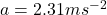.

Explanation:

The expression for the average acceleration of the particle is as follows;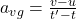Here,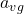is the average acceleration, v is the final speed, u is the initial speed, t is the initial time and t’ is the final time.

It is given in the problem that at a certain time a particle had a speed of 46 m/s in the positive x-direction, and 9.1 s later its speed was 67 m/s in the opposite direction.

Put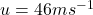,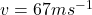, t= 0 and t’=9.1 s in the above expression.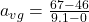Therefore, the average acceleration of the particle during this 9.1 s interval is.